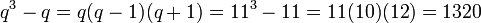# Special linear group:SL(2,11)

View a complete list of particular groups (this is a very huge list!)[SHOW MORE]

## Definition

This group is defined as the special linear group of degree two over field:F11.

## Arithmetic functions

Want to compare and contrast arithmetic function values with other groups of the same order? Check out groups of order 1320#Arithmetic functions
Function Value Similar groups Explanation
order (number of elements, equivalently, cardinality or size of underlying set) 1320 groups with same order As$SL(2,q)$,$q = 11$:$q^3 - q = q(q-1)(q+1) = 11^3 - 11 = 11(10)(12) = 1320$
number of conjugacy classes 15 groups with same order and number of conjugacy classes | groups with same number of conjugacy classes As$SL(2,q)$,$q = 11$ (odd):$q + 4 = 11 + 4 = 15$

## GAP implementation

### Group ID

This finite group has order 1320 and has ID 13 among the groups of order 1320 in GAP's SmallGroup library. For context, there are groups of order 1320. It can thus be defined using GAP's SmallGroup function as:

SmallGroup(1320,13)

For instance, we can use the following assignment in GAP to create the group and name it$G$:

gap> G := SmallGroup(1320,13);

Conversely, to check whether a given group$G$ is in fact the group we want, we can use GAP's IdGroup function:

IdGroup(G) = [1320,13]

or just do:

IdGroup(G)

to have GAP output the group ID, that we can then compare to what we want.

### Other descriptions

Description Functions used
SL(2,11) SL